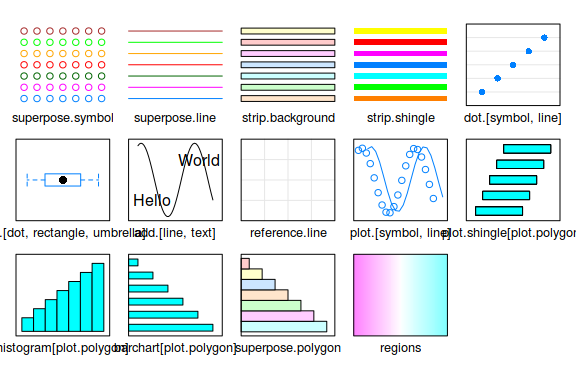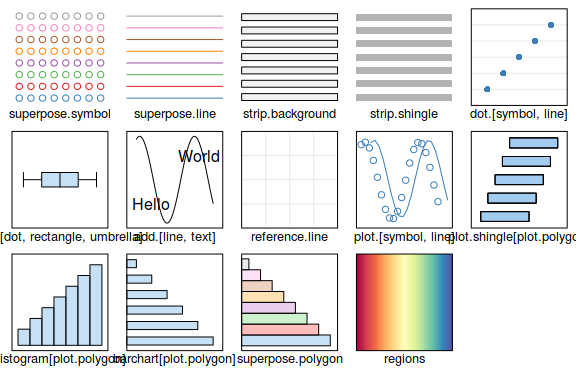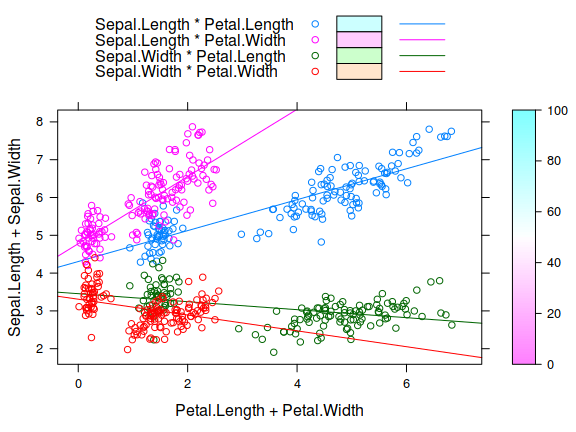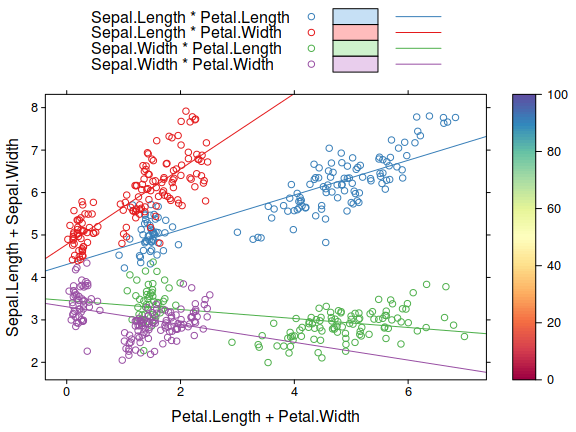# The tactile theme

### tactile

#### 2018-04-23

tactile introduces a new theme for latice that does a few things, namely

• minimizes paddings around the plot,
• halves axis tick lengths,
• uses a color theme based on RColorBrewer,
• decreases title size slightly,
• Sets strip backgrounds to grey.

We now turn to illustrating the differences. First, we use lattice::show.settings() for an overview over the default settings.

library(tactile)
show.settings()
show.settings(tactile.theme())Then we plot a sample plot (from http://latticeextra.r-forge.r-project.org/).

set.seed(0)

## create a plot to demonstrate graphical settings
obj <- xyplot(Sepal.Length + Sepal.Width ~ Petal.Length + Petal.Width, iris,
type = c("p", "r"), jitter.x = TRUE, jitter.y = TRUE, factor = 5,
auto.key = list(lines = TRUE, rectangles = TRUE))
obj <- update(obj,
legend = list(right = list(fun = "draw.colorkey",
args = list(list(at = 0:100)))))

## draw with default theme
obj## draw with tactile.theme()
update(obj, par.settings = tactile.theme())